# Electric potential energy and electric potential are the

• Slides: 11Electric potential energy and electric potential are the same thing. A. Yes B. No C. Sometimes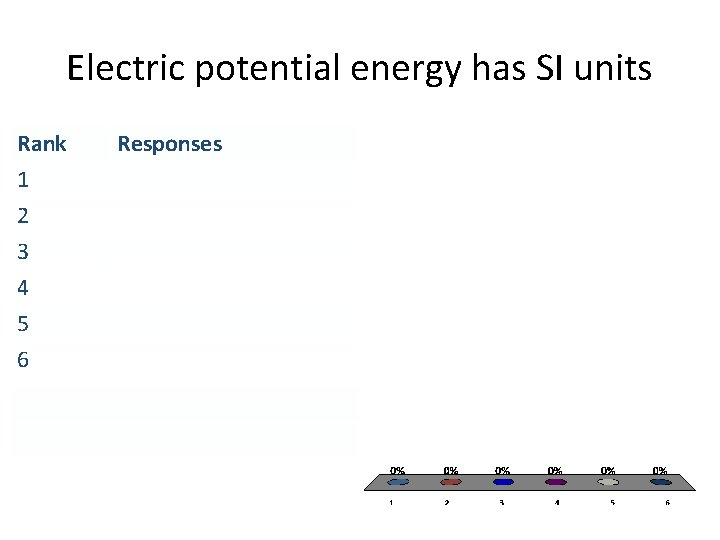Electric potential energy has SI units Rank 1 2 3 4 5 6 ResponsesElectric potential has SI units Rank 1 2 3 4 5 6 Responses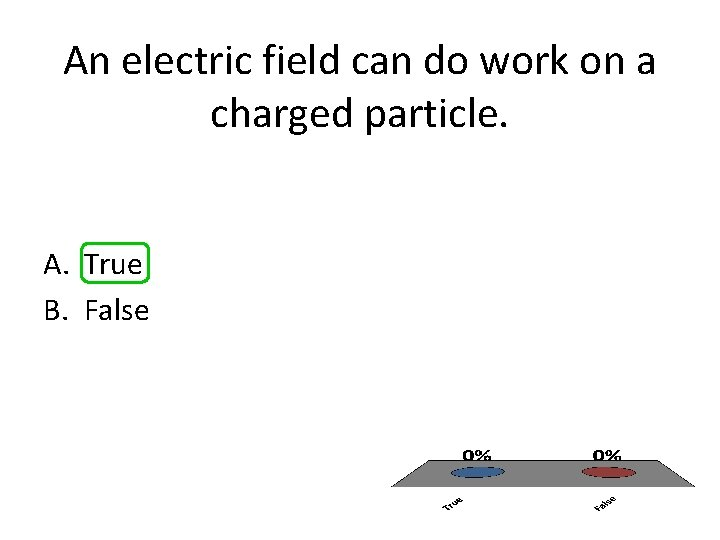An electric field can do work on a charged particle. A. True B. False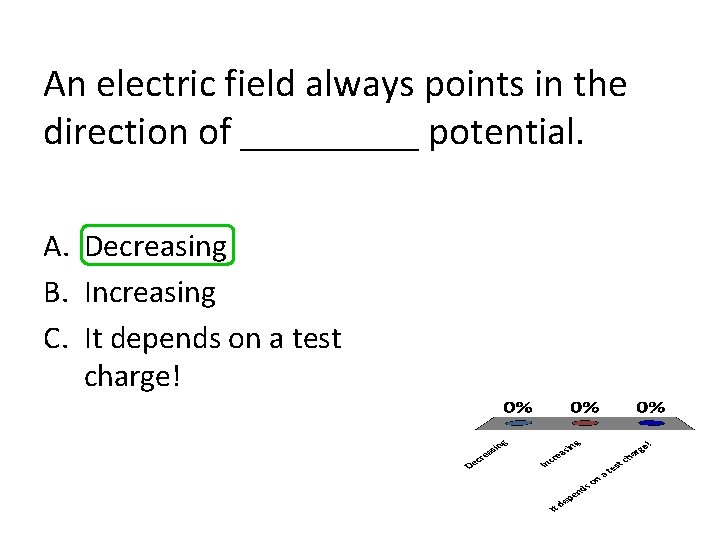An electric field always points in the direction of _____ potential. A. Decreasing B. Increasing C. It depends on a test charge!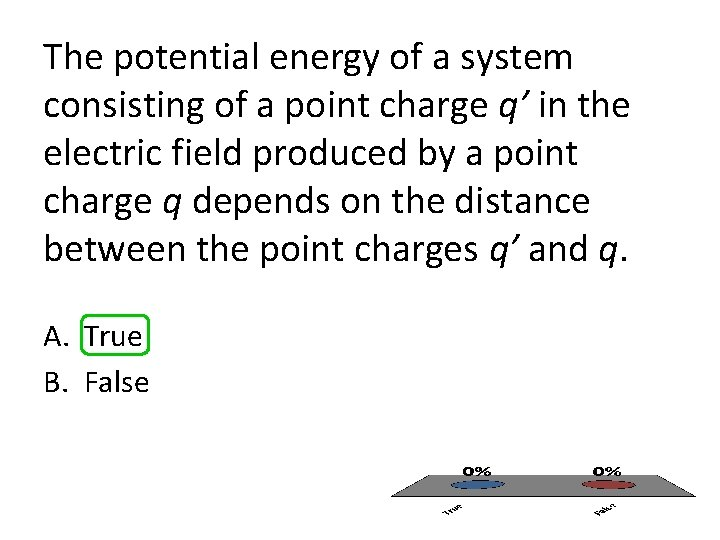The potential energy of a system consisting of a point charge q’ in the electric field produced by a point charge q depends on the distance between the point charges q’ and q. A. True B. FalseW = F*d and F = E*q implies that W = d*E*q for a particle in a constant electric field. A. True B. False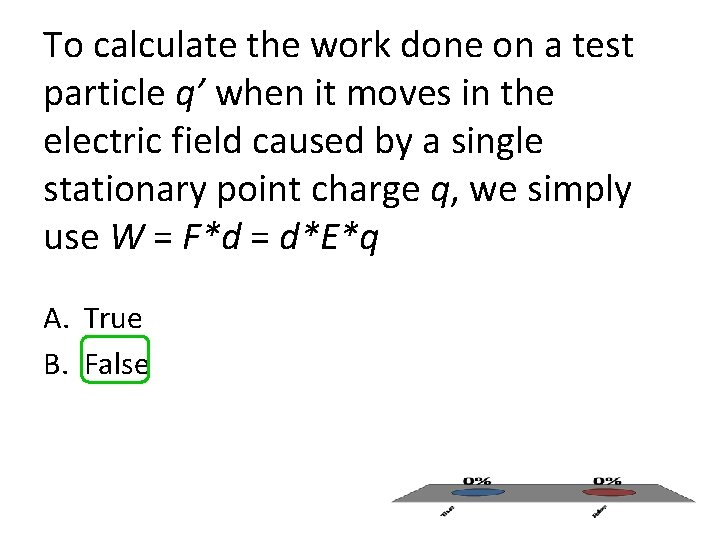To calculate the work done on a test particle q’ when it moves in the electric field caused by a single stationary point charge q, we simply use W = F*d = d*E*q A. True B. FalseThe field in the figure to the right is uniform, and both particles are moving to the right. The force exerted by the field on the positively and negatively charged particles is, points respectively, A. B. C. D. to the right, to the left, to the right to the left, to the left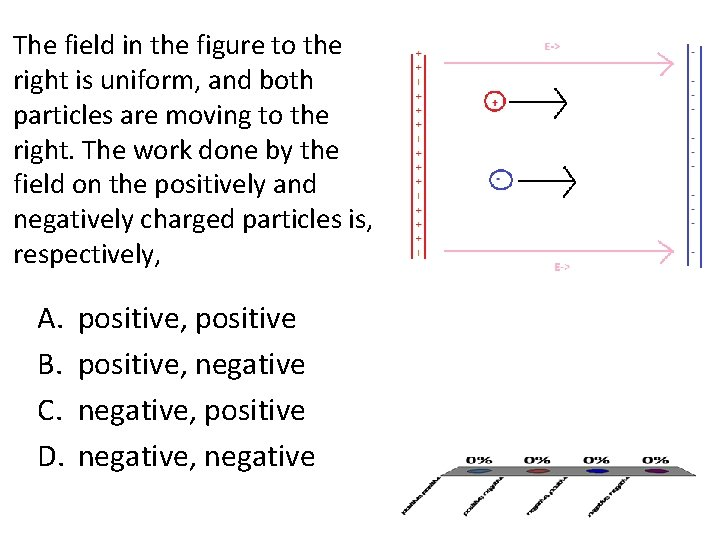The field in the figure to the right is uniform, and both particles are moving to the right. The work done by the field on the positively and negatively charged particles is, respectively, A. B. C. D. positive, negative, positive negative, negative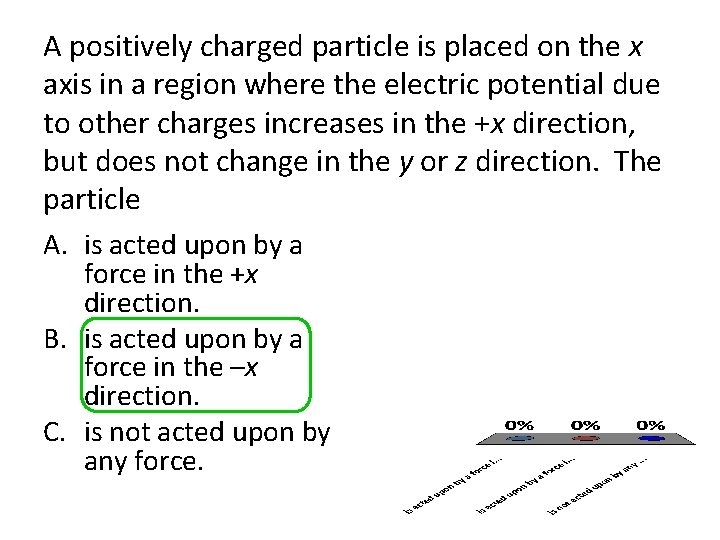A positively charged particle is placed on the x axis in a region where the electric potential due to other charges increases in the +x direction, but does not change in the y or z direction. The particle A. is acted upon by a force in the +x direction. B. is acted upon by a force in the –x direction. C. is not acted upon by any force.